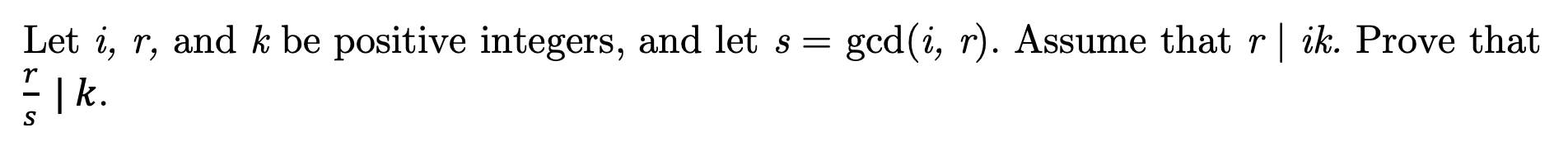# Let i, r, and k be positive integers, and let sgcd(i, r). Assume that r | ik. Prove that| k.

Question
9 viewshelp_outlineImage TranscriptioncloseLet i, r, and k be positive integers, and let s gcd(i, r). Assume that r | ik. Prove that | k. fullscreen
check_circle

Step 1

To prove the divisibility property under the given conditions

Step 2

To prove the given statement , we use the Euclidean algorithm: The gcd s of i and r...

### Want to see the full answer?

See Solution

#### Want to see this answer and more?

Solutions are written by subject experts who are available 24/7. Questions are typically answered within 1 hour.*

See Solution
*Response times may vary by subject and question.
Tagged in

### Math